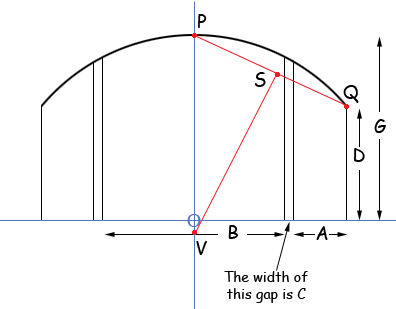I need to calculate Dimension E and F. I am given A, B, C, (or over all A+B+C), D, G. The radius is one continuous unknown radius. Example: A = 23.50” B = 35.50” C = 0.50” D = 11.50” G = 23.50” I have calculated this for angles but my mind is drawing a blank for the radius calculation. I can draw it but I need to put into Excel spreadsheet. Thanks for your assistance, Kaye Hi Kaye, I redrew the diagram you sent based on out email conversation, and labeled some points.I drew a coordinate axis with the origin at O, the midpoint of the line of length B. V is the centre of the circle and lies of the y-axis. Since P and Q are on the circle the centre must lie on the right bisector of the line segment PQ. Hence I am going to find the equation of this right bisector and see where it crosses the y-axis. This will give me the centre and radius of the circle from which you should be able to determine the other dimensions you need. P has coordinates (0,G) and Q has coordinates ( B/2 + C + A, D) and hence S the midpoint of PQ has coordinates ( (B + 2C + 2A)/4 , (D + G)/2) The line through P and Q has slope  (2D - 2G)/(B + 2C + 2A) and thus the right bisector of the line segment PQ has slope  (B + 2C + 2A)/(2G - 2D) Thus the right bisector of the line segment PQ has equation y - (D + G)/2 =  (B + 2C + 2A)/(2G - 2D) (x -  (B + 2C + 2A)/4 ) This line intersects the y-axis at V which has x-coordinate 0 so the y-coordinate of V is y  =  (B + 2C + 2A)/(2G - 2D) (0 -  (B + 2C + 2A)/4 ) +  (D + G)/2 = - (B + 2C + 2A)2/(8G - 8D) +  (D + G)/2 With the example dimensions you sent I got V to have coordinates (0, -55.12) and hence the radius of the circle is 23.5 + 55.1 = 78.6". Harley